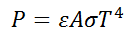# Radiation Energy using Stefan-Boltzmann Law Calculator

This CalcTown calculator calculates the radiation energy emitted by any surface above Absolute Zero using Stefan-Boltzmann's Law.

m2
K

#### Result

Wwhere

P: Radiation Energy
ε: Emissivity of the Surface. Check emissivity table below of common materials.
A: Surface Area, in m^2.
σ: Stefan-Boltzmann Constant=5.67 × 10-8 W/m2•K4
T: Absolute Temperature